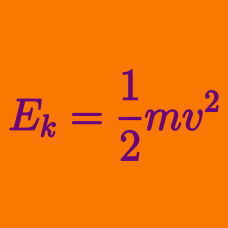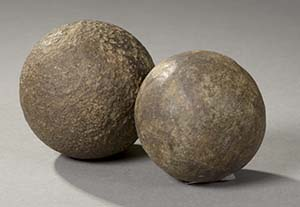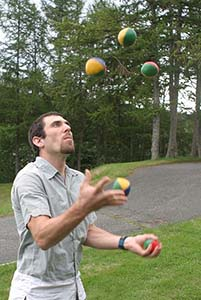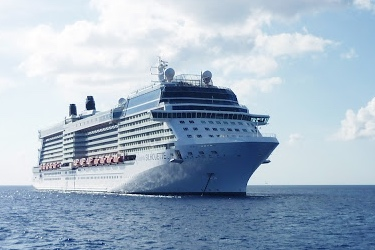Classical Mechanics

Kinetic Energy WarmupTwo cannonballs have equal mass. If the first cannonball has greater momentum, then which cannonball has greater kinetic energy?A brick is tied to a rope and is whirled in a circular path such that the brick moves with constant speed. How does the kinetic energy of the brick change with time?When a ball is thrown up into the air, how does its kinetic energy change with time?Two trucks of equal mass are moving towards each other at equal speeds.

• One of the truck drivers calculates the total kinetic energy of the trucks, in her frame.

• A person standing on the ground also calculates the total kinetic energy of the trucks, in his frame.

Who has calculated a greater value of kinetic energy?If the speed of a ship doubles, then what happens to its kinetic energy?

×